# Consider the decomposition of a metal oxide to its elements, where M represents a generic metal

Consider the decomposition of a metal oxide to its elements, where M represents a generic metal.

M203(s)—> 2M(s) + 3/2 O2(g)

info given for Gf(kJ/mol):
M203= -6.70
M(s)=0
O2(g)= 0

what is the standard change in Gibbs energy for rxn as written in forward direction? (kJ/mol)
What is the equilibrium constant (K) of this rxn, as written in forward direction at 298K?

What is the equilibrium pressure of O2(g) over M(s) at 298K? (atm)

Concepts and reason

• The difference between free energy of products and free energy of reactants is represented as standard free energy change of the reaction.
• The ratio of concentration of a product to the ratio of concentration of a reactant is represented as equilibrium constant. The equilibrium constant is expressed as.
• The equilibrium constant, in terms of partial pressure, is represented as equilibrium pressure.

Fundamentals
The standard Gibb’s free energy change can be calculated by: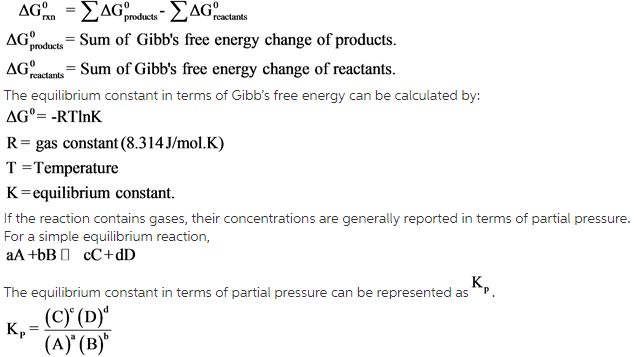Given reaction :The Gibb’s free energy change of products and reactants were given. Gibb’s free change for reaction in forward direction can be calculated by substituting the given values in Gibb’s free energy formula.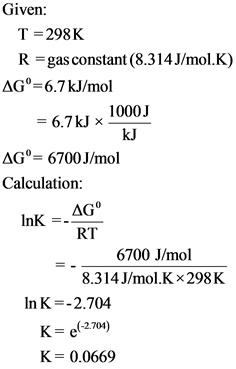From the given temperature, the equilibrium constant K is calculated by substituting the given values in Gibb’s free energy formula with respect to equilibrium constant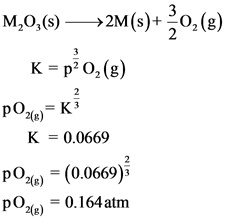The standard change in Gibb’s free energy for the reaction in forward direction is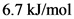.
The equilibrium constant K of this reaction is 0.0669.
The equilibrium pressure of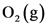over M(s) at 298K is 0.164 atm.

From the given reaction, only oxygen is gas. Therefore, the equilibrium pressure of oxygen is calculated in terms of partial pressure using equilibrium constant value.